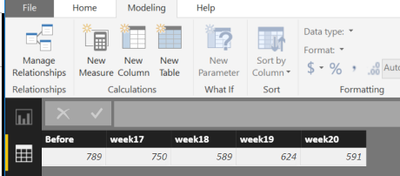cancel
Showing results for
Did you mean:

Fabric is Generally Available. Browse Fabric Presentations. Work towards your Fabric certification with the Cloud Skills Challenge.Helper I

## Transpose with DAX

Hi all,

I have calculated new columns (week17-week20) with DAX formulas from another table ('Excel').

( week20 = Table1[week19]+CALCULATE(COUNTA('Excel'[Week]);'Excel'[Week]=20;'Excel'[State]="Open")-CALCULATE(COUNTA('Excel'[Week]);'Excel'[Week]=20;'Excel'[State]="Closed")These numbers represent the amount of Open workorders at the end of the week. I would like to draw curve with these values (axis=weeks, values=open workorders), but to do that I have to transpose the table into two columns. Is it possible to do that with DAX? I can't do it with power query because the DAX-calculated valued doesn't show there.

Thank's, Oskari

6 REPLIES 6Microsoft

Hi @OskariNi,

These numbers represent the amount of Open workorders at the end of the week. I would like to draw curve with these values (axis=weeks, values=open workorders), but to do that I have to transpose the table into two columns. Is it possible to do that with DAX?

You can new an other calculated table using UNION() function.

```NewTable =
UNION (
SELECTCOLUMNS ( Table1, "Week", "Before", "Value", Table1[Before] ),
SELECTCOLUMNS ( Table1, "Week", "week17", "Value", Table1[week17] ),
SELECTCOLUMNS ( Table1, "Week", "week18", "Value", Table1[week18] ),
SELECTCOLUMNS ( Table1, "Week", "week19", "Value", Table1[week19] ),
SELECTCOLUMNS ( Table1, "Week", "week20", "Value", Table1[week20] )
)```

Best regards,

Yuliana Gu

Community Support Team _ Yuliana Gu
If this post helps, then please consider Accept it as the solution to help the other members find it more quickly.Regular Visitor

Thank youMicrosoft

This calculated table might get close

```Table =
SUMMARIZECOLUMNS(
'Excel'[Week],
"Value",
CALCULATE(COUNTA('Excel'[Week]),'Excel'[State]="Open")
-
CALCULATE(COUNTA('Excel'[Week]),'Excel'[State]="Closed")
)```

Proud to be a Datanaut!Helper I

It's close but the problem is that I need to use the situation of the previous week as a starting point for the next week. This solution gives me the difference between closed and opened per week not the amount of open workorders.

OskariMicrosoft

Is this better?

```Table =
SUMMARIZECOLUMNS(
'Excel'[Week],
"Value",
VAR x = MIN('Excel'[Week])
RETURN
CALCULATE(COUNTA('Excel'[Week]),'Excel'[State]="Open",'Excel'[Week]=x )
-
CALCULATE(COUNTA('Excel'[Week]),'Excel'[State]="Closed",'Excel'[Week]=x-1 )
)```

Proud to be a Datanaut!Helper I

This way below it works for the 2 first weeks (17 and 18) but then 19 is wrong because it doesn't take into account week 17 (it requires a cumulative sum). It would be really easy if I just could flip the row to form a column but I guess that is not possible?

Table2 = SUMMARIZECOLUMNS(

‘Excel’[Week];

"Value";        VAR x = MIN(‘Excel’[Week])

RETURN

CALCULATE(COUNTA(‘Excel’[Week]);’Excel’[State]="Open";’Excel’[Week]=x)

-

CALCULATE(COUNTA(‘Excel’[Week]);’Excel’[State]="Closed";’Excel’[Week]=x)

+

CALCULATE(COUNTA(‘Excel’[Week]);’Excel’[State]="Open";’Excel’[Week]=x-1)

-

CALCULATE(COUNTA(‘Excel’[Week]);’Excel’[State]="Closed";’Excel’[Week]=x-1))Announcements#### Power BI Monthly Update - November 2023

Check out the November 2023 Power BI update to learn about new features.#### Fabric Community News unified experience

Read the latest Fabric Community announcements, including updates on Power BI, Synapse, Data Factory and Data Activator.#### The largest Power BI and Fabric virtual conference

130+ sessions, 130+ speakers, Product managers, MVPs, and experts. All about Power BI and Fabric. Attend online or watch the recordings.Top Solution Authors
Top Kudoed Authors
Users online (3,665)